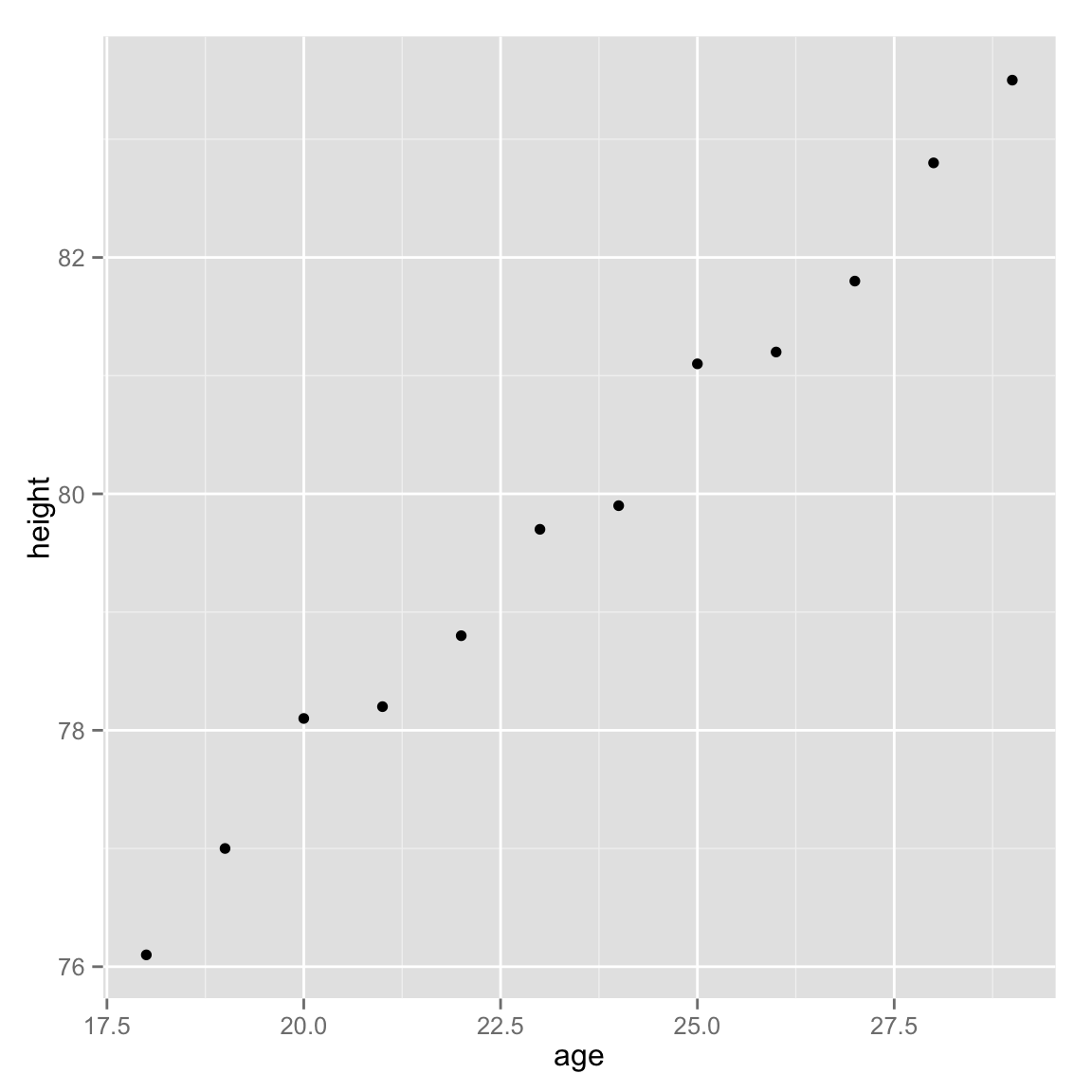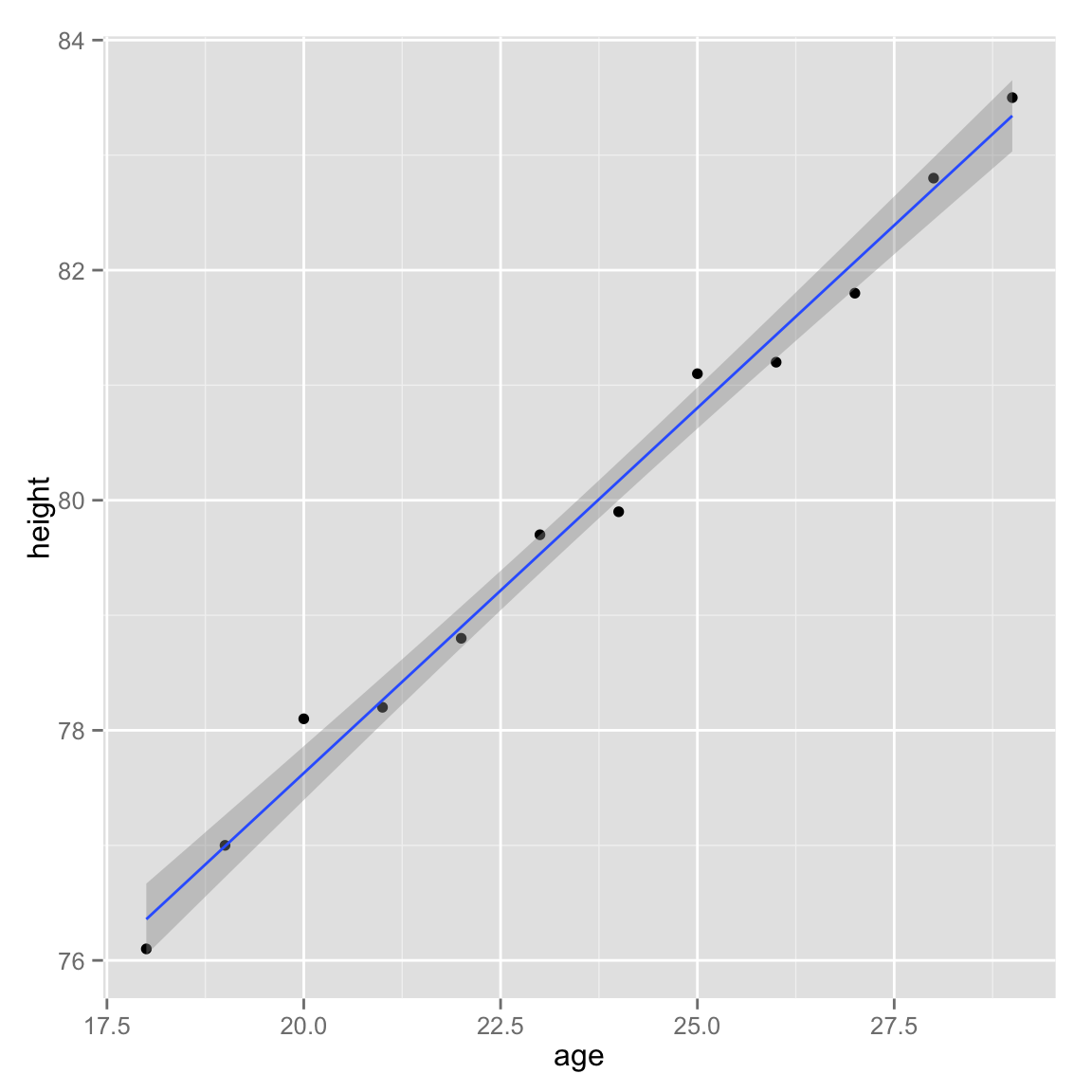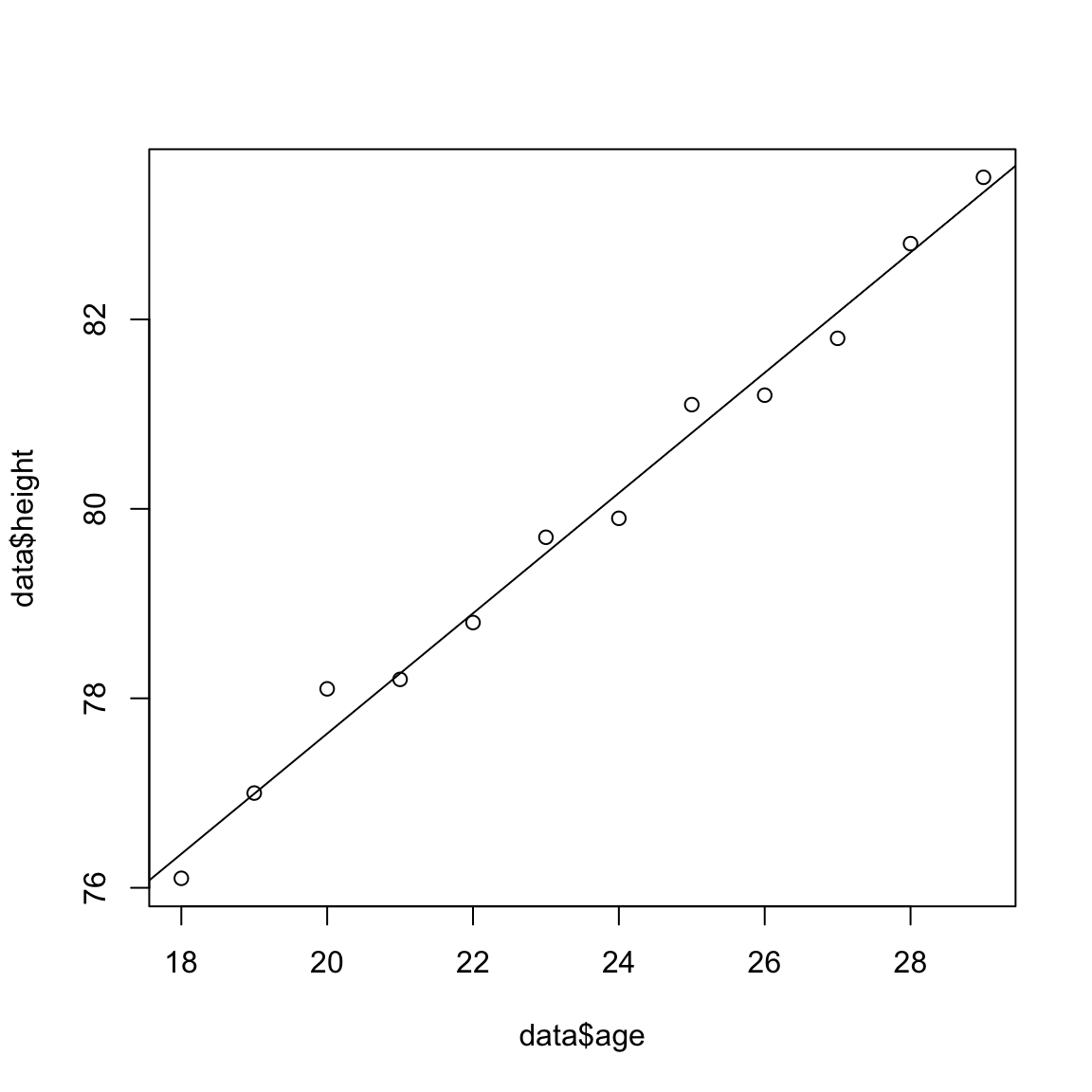When you’ve got a scatterplot you want to add a regression to, there are a number of ways.

Let’s make some data:

age = 18:29
height = c(76.1,77,78.1,78.2,78.8,79.7,79.9,81.1,81.2,81.8,82.8,83.5)

data = data.frame(age, height)

print(data)
##    age height
## 1   18   76.1
## 2   19   77.0
## 3   20   78.1
## 4   21   78.2
## 5   22   78.8
## 6   23   79.7
## 7   24   79.9
## 8   25   81.1
## 9   26   81.2
## 10  27   81.8
## 11  28   82.8
## 12  29   83.5

plot it. we’ll use a different plotting library this time, though, since it’s easier to make pretty things.

library(ggplot2)

ggplot(data, aes(x=age, y=height)) +
geom_point()Now, to get a best-fit line all we have to do it:

ggplot(data, aes(x=age, y=height)) +
geom_point() +
geom_smooth(method="lm")If we want to see how “good” this is, we can look at the lm output ourselves:

fit = lm(data$height~data$age)

print(fit)
##
## Call:
## lm(formula = data$height ~ data$age)
##
## Coefficients:
## (Intercept)     data$age ## 64.928 0.635 print(summary(fit)) ## ## Call: ## lm(formula = data$height ~ data$age) ## ## Residuals: ## Min 1Q Median 3Q Max ## -0.27238 -0.24248 -0.02762 0.16014 0.47238 ## ## Coefficients: ## Estimate Std. Error t value Pr(>|t|) ## (Intercept) 64.9283 0.5084 127.71 < 2e-16 *** ## data$age      0.6350     0.0214   29.66 4.43e-11 ***
## ---
## Signif. codes:  0 '***' 0.001 '**' 0.01 '*' 0.05 '.' 0.1 ' ' 1
##
## Residual standard error: 0.256 on 10 degrees of freedom
## Multiple R-squared:  0.9888, Adjusted R-squared:  0.9876
## F-statistic:   880 on 1 and 10 DF,  p-value: 4.428e-11

Remember, we care about R-squared and the p-value (bottom right two rows).

A good ‘fit’ is when R-squared is closer to 1 (that means your model describes the data well) and when the p-value is below 0.05. Those aren’t hard-and-fast rules, but a good rule-of-thumb to use.

You can use the other plotting system we saw last week, too:

plot(data$age, data$height)
abline(fit)it’s just not as pretty.

We can even use this model we’ve made to make a prediction. In the output of print(fit) we have coefficients that we can use in this basic regression formula. To make a prediction, we have to take the data\$age coefficient, multiply it by the age we want to predict the height for then add the intercept. so, our formula would be:

height = 0.635 * age + 64.928

So, if the age we want to predict height for is 32 then the estimate is that it’ll be:

print(0.635 * 32 + 64.928)
##  85.248

It should be nearly spot-on for existing ones, too (say, 25):

print(0.635 * 25 + 64.928)
##  80.803
print(data[age==25,])
##   age height
## 8  25   81.1

Remember, it won’t be perfect (it’s a prediction), but it’ll be close with some error margin.